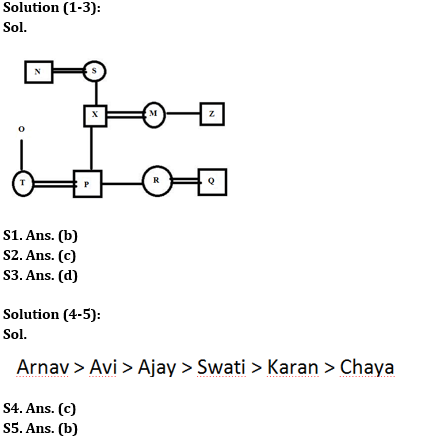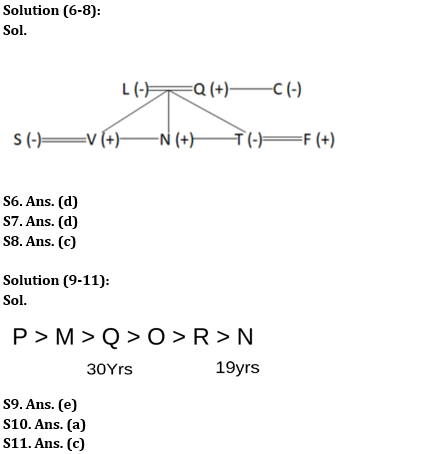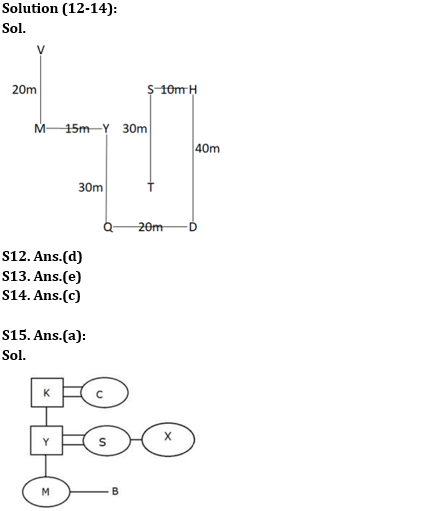Latest Banking jobs   »

# Reasoning Ability Quiz For SBI Clerk Prelims 2022- 25th May

Directions (1-3): Read the following information carefully and answer the questions given below.
A three-generation family consists of ten members i.e., N, O, X, P, R, Z, M, S, Q, and T. S is the mother of P’s father. Z who is not married to T is the maternal uncle of R. Q’s mother-in-law is the daughter-in-law of N. R who is the only sibling of P is married to Q. T does not belong to the generation of M. X is one of the male members of the family. O is not the parent of N’s daughter-in-law. N has at least one granddaughter. Husband of T is the son-in-law of O.

Reasoning Ability Quiz For SBI Clerk Prelims 2022 CHECKIN HINDI

Q1. What is the relation of M with respect to N?
(a) Son-in-law
(b) Daughter-in-law
(c) Daughter
(d) Uncle
(e) None of these

Q2. What is the relation of O with respect to P?
(a) Father-in-law
(b) Father
(c) Either Father-in-law or Mother-in-law
(d) Either Uncle or aunt
(e) Either Brother or sister

Q3. Which of the following is not the pair of father and child?
(a) N and X
(b) P and X
(c) X and R
(d) O and T
(e) None of these

Directions (4-5): Study the information given below and answer the questions based on it.
Ajay is heavier than Swati, Arnav is heavier than Avi, Karan is heavier than Chaya, Avi is heavier than Ajay and Swati are heavier than Karan.

Q4. Who is the heaviest among them?
(a) Avi
(b) Karan
(c) Arnav
(d) Swati
(e) None of these

Q5. Who is Lightest among them?
(a) Ajay
(b) Chaya
(c) Swati
(d) Karan
(e) None of these

Directions (6-8): Study the information carefully and answer the questions accordingly.
There are eight members in a family with three married couples. N is the nephew of C. S is the sister-inlaw of N. T is the wife of F and the daughter of L. Q is the father of V and N. Q has a sister. F is the brother-in-law of V.

Q6. How many females are there?
(a) Two
(b) Five
(c) Three
(d) Four
(e) None of these

Q7. How V is related to S?
(a) Son
(b) Father
(c) Brother
(d) Husband
(e) None of these

Q8. How Q is related to F?
(a) Father
(b) Nephew
(c) Father-in-law
(d) Uncle
(e) None of these

Directions (9-11): Study the following information carefully to answer the given questions.
M, N, O, P, Q, and R are six males in a family. O is not younger than R and not elder than Q. N is not elder than O. M is not younger than Q as well as not elder than P. The one who is the third eldest is 30 years old. The one who is the youngest among them is 19 years old. R is not the youngest among them.

Q9. Who among the following is possibly 20 years old?
(a) M
(b) P
(c) R
(d) O
(e) Can’t be determined

Q10. How many members are elder than Q?
(a) Two
(b) Four
(c) Five
(d) Three
(e) None

Q11. If G is the member of the family, he is not elder than Q and not younger than R, then which of the following statements is true with respect to G?
(a) Elder than M but younger than P
(b) Younger than N but elder than O
(c) Probably elder than O but younger than P
(d) He is 4th youngest
(e) 5th eldest.

Directions (12-14): Study the following information carefully to answer the given questions.
Point D is 40m south of point H and 20m east of point Q. Point S is 30m north of point T. Point M is 20m south of point V and 15m west of point Y. Point Q is 30m south of point Y. Point H is 10m east of point S.

Q12. Point M is in which direction of point T?
(a) East
(b) South-East
(c) West
(d) North-West
(e) None of these

Q13. Four from the following are similar in a certain way and forms a group. Find out the one, which does not belong to that group.
(a) Q, H
(b) Q, T
(c) Y, H
(d) M, S
(e) D, Y

Q14. Point S is in which direction of point Q?
(a) South
(b) North
(c) North-East
(d) South-West
(e) None of these

Q15. There is a family of seven members i.e., K, Y, S, M, X, B and C. K is the father of B’s father.X is B’s maternal aunt. Y is M’s father. M is B’s sister. S is married to Y.C is married to S’s father-in-law. How is K related to B?
(a) Grandfather
(b) Brother-in-law
(c) Father
(d) Brother
(e) None of these

Solutions#### Congratulations!